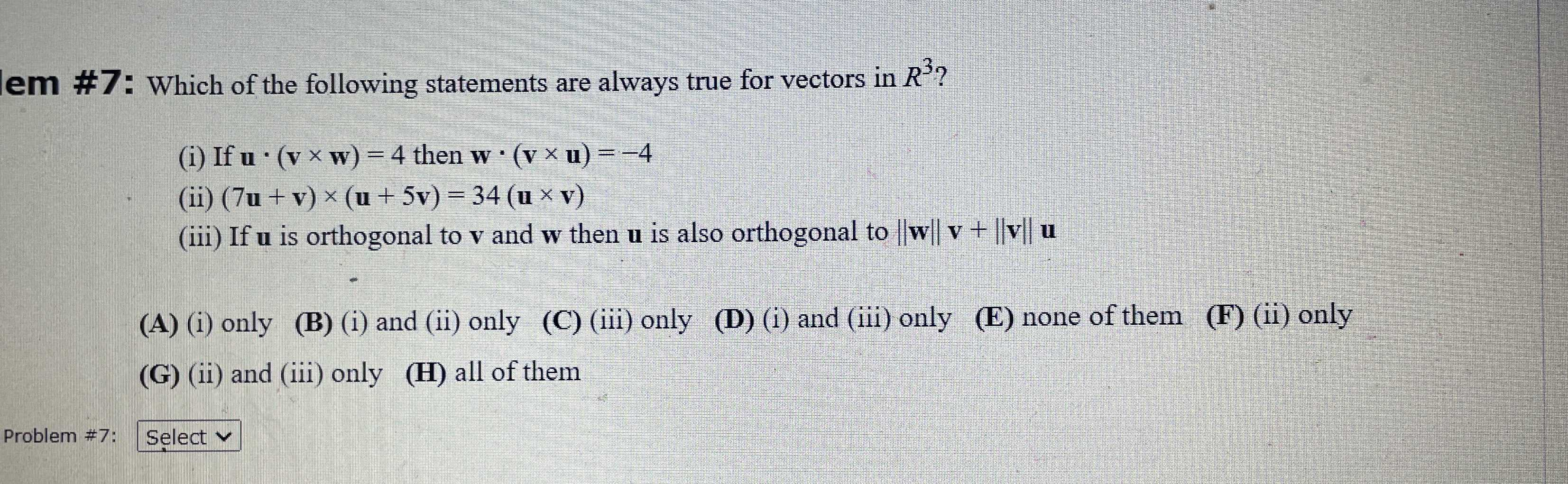### Still have math questions?

Algebra
QuestionWhich of the following statements are always true for vectors in $$R ^ { 3 }$$ ?

(i) If $$u \cdot ( v \times w ) = 4$$ then $$w \cdot ( v \times u ) = - 4$$

(ii) $$( 7 u + v ) \times ( u + 5 v ) = 34 ( u \times v )$$

(iii) If $$u$$ is orthogonal to $$v$$ and $$w$$ then $$u$$ is also orthogonal to $$\| w \| v + \| v \| u$$

(A) (i) only

(B) (i) and (ii) only

(C) (iii) only

(D) (i) and (iii) only

(E) none of them

(F) (ii) only

(G) (ii) and (iii) only

(H) all of them